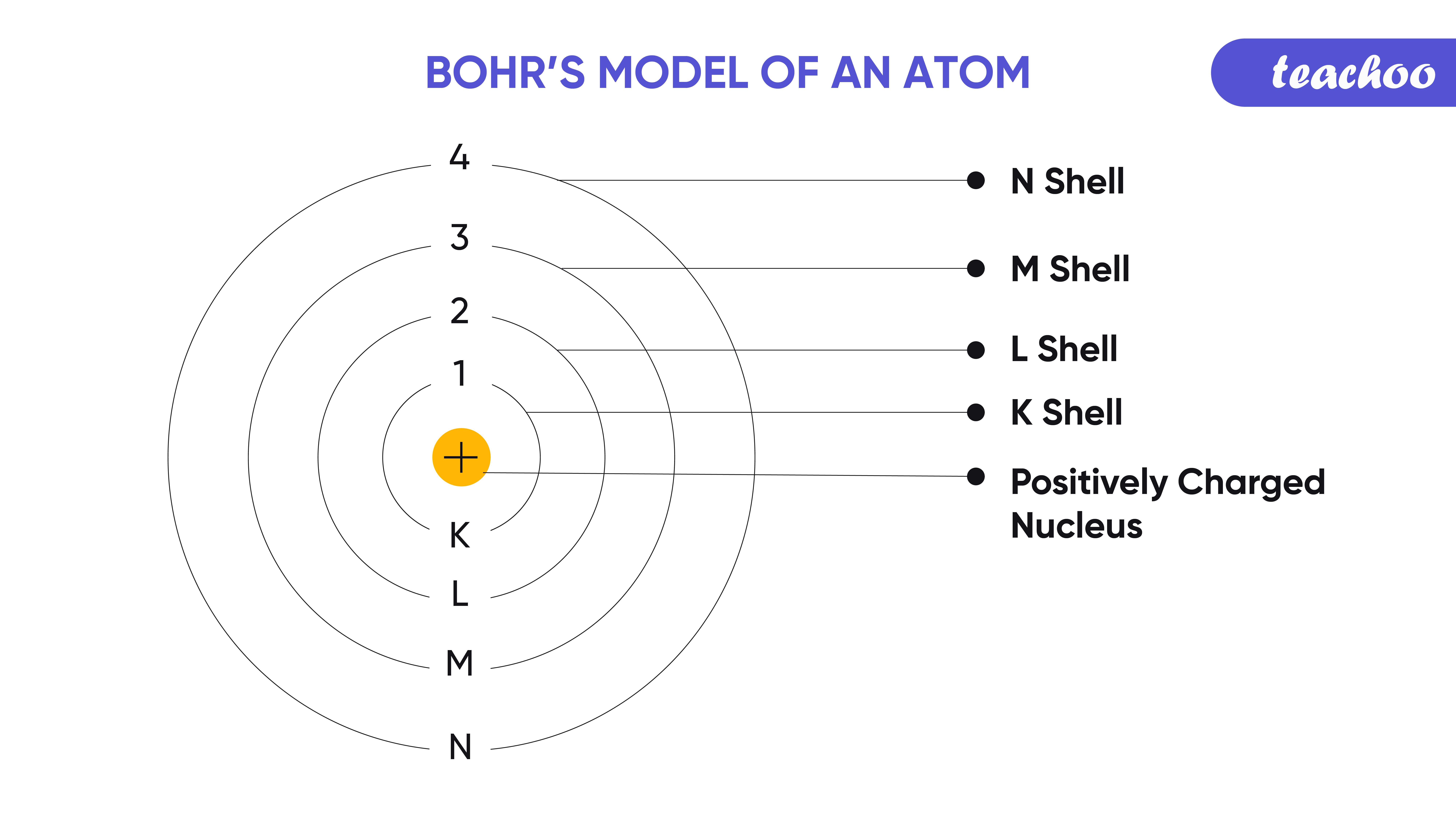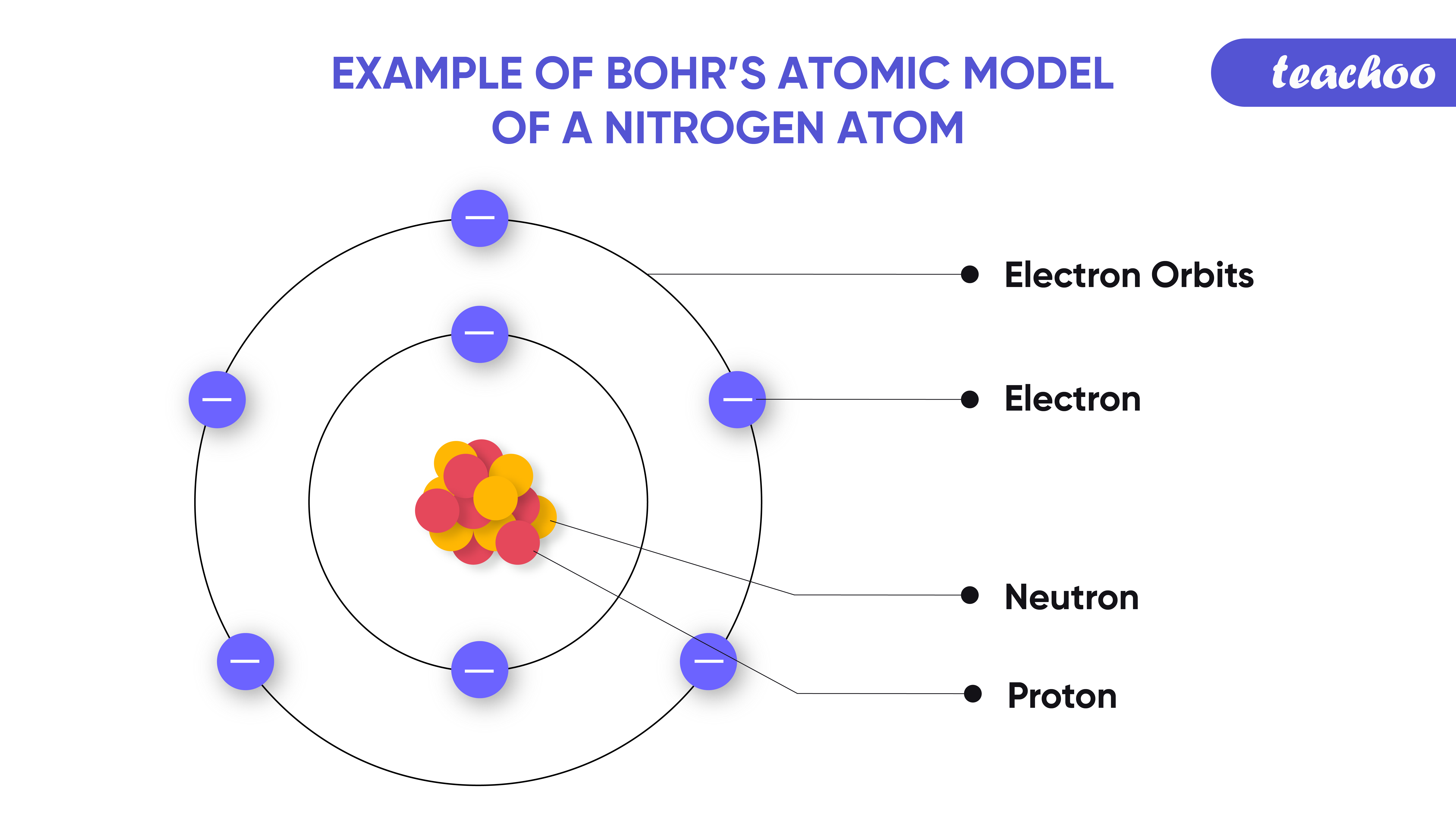This Model was proposed by the scientist Niel Bohr

As per this Model,

1. Electrons move around Nucleus of an atom.
But they move in discrete orbits (specific paths).
These orbits are called energy levels or shells.

Different Energy shells are:-

K shell, L shell, M shell and N shell .Other Name of These shells are:-

 Shell Name Other Name K shell n=1 L Shell n=2 M Shell n=3 N Shell n=4

• Each energy shells have different amount of electrons and different energy level

• Example; K shell can hold less electrons and has less energy level than the L shell and so on.

 Shell Name n Number of Electrons Energy Level K shell n = 1 2 Low Energy Level L shell n = 2 8 Medium Energy Level M shell n = 3 18 High Energy Level N shell n = 4 32 Max Energy Level2.Electrons keep on revolving in the same shell.

But they do not radiate energy while revolving in these shells (there is no loss of energy ).

Examples -

In text Question - Page 49 Q3

NCERT Back Exercise - Q5

1. Class 9
2. Chapter 4 Class 9 - Structure of Atom (Term 2)
3. Concepts

Concepts

Class 9
Chapter 4 Class 9 - Structure of Atom (Term 2)